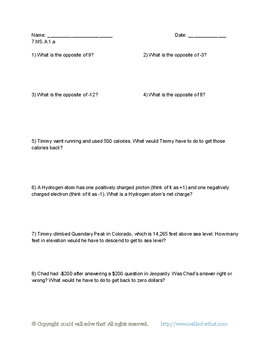# Math: 7th grade Number System 7.NS.A.1Subject
Resource Type
File Type

PDF

(177 KB|8 pages)
Product Rating
Standards
• Product Description
• StandardsNEW
This packet includes 4 worksheets of 8 problems each with an answer key provided. These worksheets cover all of Common Core standard 7.NS.A.1, with one worksheet per sub-standards 7.NS.A.1.a, 7.NS.A.1.b, 7.NS.A.1.c, and 7.NS.A.1.d.
Apply properties of operations as strategies to add and subtract rational numbers.
Understand subtraction of rational numbers as adding the additive inverse, 𝘱 – 𝘲 = 𝘱 + (–𝘲). Show that the distance between two rational numbers on the number line is the absolute value of their difference, and apply this principle in real-world contexts.
Understand 𝘱 + 𝘲 as the number located a distance |𝘲| from 𝘱, in the positive or negative direction depending on whether 𝘲 is positive or negative. Show that a number and its opposite have a sum of 0 (are additive inverses). Interpret sums of rational numbers by describing real-world contexts.
Describe situations in which opposite quantities combine to make 0. For example, a hydrogen atom has 0 charge because its two constituents are oppositely charged.
Apply and extend previous understandings of addition and subtraction to add and subtract rational numbers; represent addition and subtraction on a horizontal or vertical number line diagram.
Total Pages
8 pages
Included
Teaching Duration
N/A
Report this Resource to TpT
Reported resources will be reviewed by our team. Report this resource to let us know if this resource violates TpT’s content guidelines.